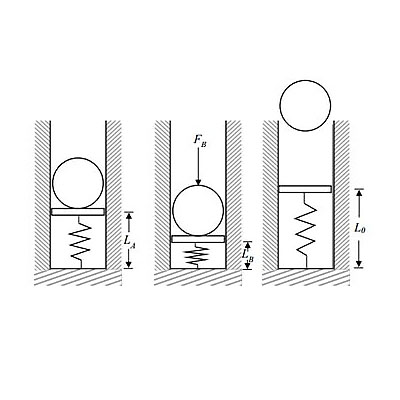# A 2.5 kg spherical projectile is launched vertically from a spring loaded gun barrel. Initially...

## Question:

A 2.5 kg spherical projectile is launched vertically from a spring loaded gun barrel. Initially at time {eq}t_A {/eq}, the projectile rests on the spring loaded plate, a distance {eq}L_A {/eq} from the bottom of the barrel. At time {eq}t_B {/eq} a constant force is applied to the projectile to compress the spring to a distance of {eq}L_B {/eq} = 0.1 m from the bottom of the barrel. At time {eq}t_C {/eq}, the force is instantaneously removed and the projectile is launched from rest vertically upward. The stiffness of the spring is {eq}k {/eq} = 180 N/m and the un-stretched length of the spring is {eq}L_0 {/eq} = 0.5 m.

Determine:

a) the length of the spring at time {eq}t_A, L_A {/eq},

b) the magnitude of the applied force {eq}F_B {/eq},

c) the change in height experienced by the projectile from {eq}t_B {/eq} until it instantaneously comes to rest, {eq}Ah {/eq}.## Spring Constant:

The force which is acting at the unit length of the spring is known as the spring constant. Generally, the spring constant is represented by letter k and expressed in terms of Newton per meter.

## Answer and Explanation: 1

Become a Study.com member to unlock this answer! Create your account

Given data

• The mass of projectile is: {eq}m = 2.5\;{\rm{kg}} {/eq}
• The length {eq}{L_B} {/eq} is: {eq}{L_B} = 0.1\;{\rm{m}} {/eq}
• The length...

See full answer below.

#### Learn more about this topic:Practice Applying Spring Constant Formulas

from

Chapter 17 / Lesson 11
3.4K

In this lesson, you'll have the chance to practice using the spring constant formula. The lesson includes four problems of medium difficulty involving a variety of real-life applications.